# Characteristic Property 1 (AAA Similarity)

## Triangles of Class 10

### THEOREM 3:

If in two triangles, the corresponding angles are equal, then the triangles are similar.

Given: Two triangles ABC and DEF in which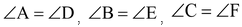.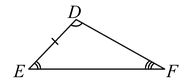To prove: ΔABC ~ ΔDEF.

Proof:

Case 1: When AB = DE

In triangles ABC and DEF, we have

∠A = ∠D [Given]

AB = DE [Given]

∠B = ∠E [Given]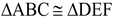[By ASA congruency]

⇒ BC = EF and AC = DF [c.p.c.t.]

Thus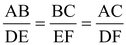[corresponding sides of similar Δs are proportional]

Hence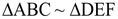Case 2: When AB < DE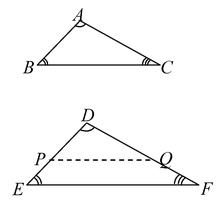Let P and Q be points on DE and DF respectively such that DP = AB and DQ = AC. Join PQ.

In ΔABC and ΔDPQ, we have

AB = DP [By construction]

∠A = ∠D [Given]

AC =DQ [By construction]

∴ ΔABC ≅ ΔDPQ [By SAS congruency]

∴ ∠ABC = ∠DPQ [c.p.c.t.] …(i)

But ∠ABC = ∠DEF [Given] …(ii)

∴ ∠DPQ = ∠DEF [c.p.c.t.]

But ∠DPQ and ∠DEF are corresponding angles.

⇒ PQ || EF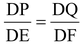[Corollary to BPT Theorem]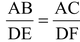. [∴ DP = AB and DQ = AC (by construction)]

Similarly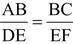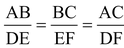.

Hence, ΔABC ~ ΔDEF.

 Case 3: When AB > DE Let P and Q be points on AB and AC respectively such that AP = DE and AQ = DF. Join PQ. In ΔAPQ and ΔDEF, we have AP = DE [By construction] AQ = DF [By construction] ∠A = ∠D [Given]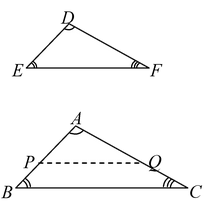∴ ΔAPQ ≅ ΔDEF [By SAS congruency]

∴ ∠APQ = ∠DEF [c.p.c.t.] …(i)

But ∠DEF = ∠ABC. [Given] …(ii)

From (i) and (ii) we have

∴ ∠APQ = ∠ABC.

But ∠APQ and ∠ABC are corresponding angles

∴ PQ || BC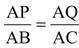[Corollary to BPT Theorem]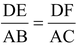[∴ AP = DE and AQ = DF (by construction)]

Similarly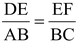.

Thus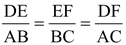Or,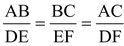.

Hence ΔABC ~ ΔDEF.